## Laser Interferometry and Gravitational Waves (ART364E)

The Physics Nobel Prize in 2017 was given to three researchers (Rainer Weiss, Barry C. Barish and Kip S. Thorne) who working on LIGO (*) were able to detect gravitational waves, provided by Einstein's theory of relativity. In this article, we are looking for a simple way to explain to our readers that they have much more knowledge about electronics than physics, to explain a little more about what was done in the experiment that led to the detection of gravitational waves.

(*) Ligo is the acronym for Laser Interferometer Gravitational-Wave Observatory

Translated into English by Patricia Lima Carlos.Rainer Weiss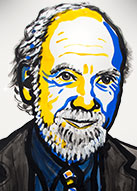Harry C. BarishKip S. Thorne

We begin by explaining what a Laser Interferometry is.

## Laser Interferometry

The interference phenomenon is widely studied in the physics of waves, being analyzed with all kinds of waves, such as sound waves, radio waves, light waves (electromagnetic waves). In our basic electronic course we detail this phenomenon, for which we give a brief explanation that aims to facilitate the understanding of this article.

a) Interference

We can take as an example the sounds that are waves with points of greater intensity and less intensity (compression and decompression). If we have two sound sources of the same frequencies, in propagation there will be points at which the compression peaks of the waves of a source coincide with the points of least compression. At these points, the canceling of the signals occurs, that is, we will have a destructive interference, as shown in Figure 1.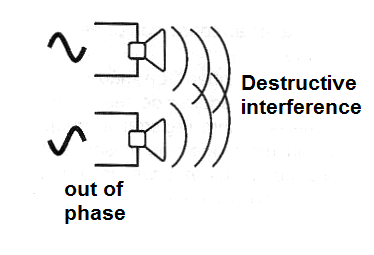Figure 1

On the other hand, if the movement of the cones is such that we have air compression in the same half-cycles. The reproduction yield will be much higher, see example in Figure 2.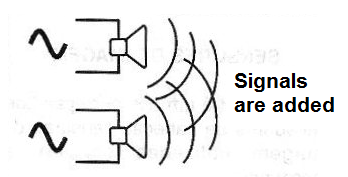Figure 2

With light waves (electromagnetic waves) the same occurs. If waves from two sources are incidenting on a screen in phase, that is, with the maximum points coinciding, they add up and in this place we will have more clarity.

If the waves are in phase opposition, the cancellation occurs. This phenomenon was used to prove the undulatory nature of light. Obtaining two beams of light in phase from a source, by passing through two holes, at the bulkhead we obtained a pattern of light and dark as shown in Figure 3, as was the cancellation or the sum of the light intensity.Figure 3

At the dark parts we have the light cancellation because the beams which produce this image are in phase opposition and in the light parts the beams are in phase adding their light.

b) Laser

The idea of the LASER, which the reader will be able to analyze in detail in article in this site, is to obtain an emission of concentrated light beam and in phase.

We have the possibility of obtaining, with laser, light in phase. The production of light under conditions of a resonant chamber, in which is obtained a standing wave, causes the emission of a laser occurs in phase, which does not happen with a common source, even though monochromatic, such as a neon or LED, where the radiation of the same wavelength appears disordered in phase. (Figure 4)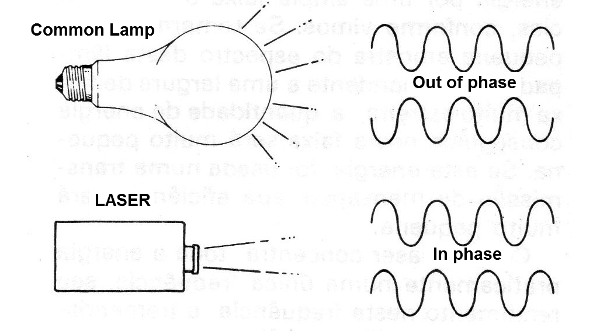Figure 4 - Emission in phase

This means that LASER is an ideal source of light in phase that can be used in physics experiments, such as the one that occurs in interferometry, which we will explain later.

First let's deal with gravitational waves.

## Gravitational waves

In physics, the influences that bodies have on each other may have several natures. These influences are called "fields."

Thus we say that a field manifests itself around a body when this body can somehow exert an influence over another body that is immersed in that field.

We who work with electricity are familiar with the electric field which manifests itself around a charged load and which can exert a force of attraction or repulsion on another load which is in it. (Figure 5)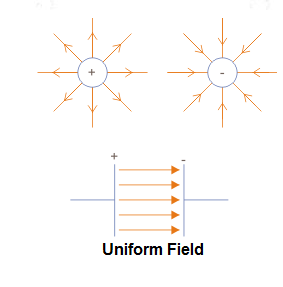Figure 5

We represent this field by force lines.

We also deal with magnetic fields, such as those produced by magnets that cause forces of attraction or repulsion to manifest on bodies that are immersed in them. (Figure 6)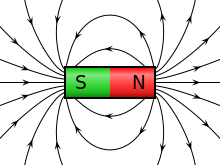Figure 6 - Magnetic field of a permanent magnet

However, there is yet another field, which is not of an electrical nature and which is present in our world.

The earth, with its great mass, creates around itself a field which acts on objects that also have mass, exerting a force of attraction. This is the gravitational field, or gravity.

As we can see in Figure 7, it is represented in the same way as an electric field of a spherical charge, except that it exerts only attraction.

Any material object placed in this field is attracted to the earth.Figure 7

Einstein, in his theory of relativity, proposed that this field would manifest exactly like the fields produced by electric charges.

When we move a charge, it produces a disturbance by creating a wave which propagates through space at the speed of light.

In fact, the light itself is created this way. A disturbance of the electric and magnetic field that propagates through space.

According to Einstein, the movement of a mass, or the annihilation of a mass, when it occurs when a star explodes as a supernova or when two black holes swallow, would produce a gravitational perturbation that would propagate through space at the speed of light.

This means that if a gigantic mass is annihilated at a particular point in the universe, its gravitational influence on a distant body would not reach it instantly, but some time later when it is struck by the disturbance generated, that is, by the gravitational wave.

Einstein thought that these waves could never be detected, for they would be extremely weak.

Even a pair of black holes with dimensions of thousands of earths, when annihilated produced a gravitational perturbation that would displace the bodies here in the earth of the order of fraction of the width of an atom.

What happens is that these waves affect what is called the space-time continuum that is the environment in which we are immersed in our universe.

Thus, it is as if the tissue of which the universe is made is flexible and can suffer contractions and strains caused by gravitational waves.

If a gravitational wave strikes an object it contracts and distends itself in an extremely small fraction, changing its dimensions.

The idea of ??detecting these waves comes precisely from this fact, coming since 1957 when it was proposed by important physicists of the time.

However, the displacements or changes of form which occur in a body through the passage of an eventual wave are so small that since that time it was not possible to make its detection.

With the development of technology this has become possible now as we come to see.

## Interferometry and LIGO

We can use a beam of light in phase to measure extremely small distances or very small displacements.

Since the wavelength of light is very small, on the order of nanometer or near the dimensions of an atom, if one of two beams of light in phase is displaced by a distance of that order, it can already leave the phase and this can be detected by sensors.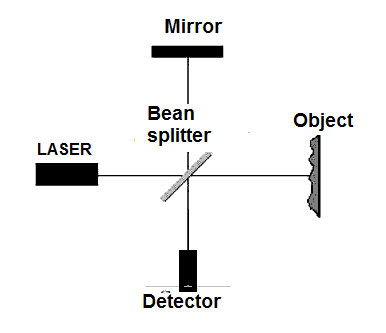Figure 8

If we make an assembly like the one shown in Figure 8 we will have a way of detecting extremely small displacements of an object.

How is this possible?

We produce a beam of LASER which, as we explained, consists of coherent light.

The beam focus on a semi transparent object, that is, an object which reflects 50% of light and allows the other 50% to pass, obtaining two LASER beams, but in directions which form a 90 degree angle.

One of the beams goes to an object and comes back, passing through the semi transparent mirror and focusing on the detector.

The other beam goes to the object where there is a trapped mirror in which it reflects and comes back. In the way back, the beam is deflected and also focus on the detector.

If the distance to the object or even the mirror is carefully adjusted we can cause the beams to arrive in phase opposition and cancel themselves.

The result will be a null signal in the detector, or the absence of light.

However, if the object moves, whether it is a motion of the order of a fraction of the dimensions of an atom, that is enough to change the phase of one of the light beams and we will have a signal in the detector.

Of course, all this must be done in an extremely delicate and precise set-up.

For this it was created within a series of experiments called LIGO an equipment or detector at two places, Hanford and Linvingston, separated by a distance of 3002 kilometers, so that, at the speed of light, we have a difference of 10 ms between the action of a possible gravitational wave in two sensors placed at these places.

The sensors are no more than two 40 kg silica mirrors in order to reflect the beam of a 20 W Nd: YAG laser from an input power of 700 W. (Figure 9)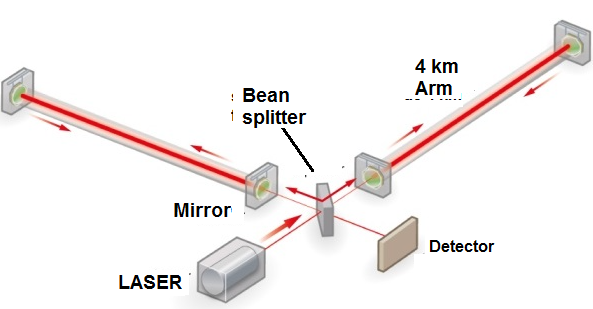Figure 9

The idea of ??two detectors at very distant places is to avoid that the detected signals are deceptive, of a near phenomenon, for example. Simultaneous detection at distant places would indicate that the disturbance actually came from the cosmos.

And, that is what happens according to the records in Figure 10.

The peaks of the signals show at the instant which a disturbance was picked up simultaneously on the two sensors by taking the phase opposition signals and thus obtaining a large amplitude response.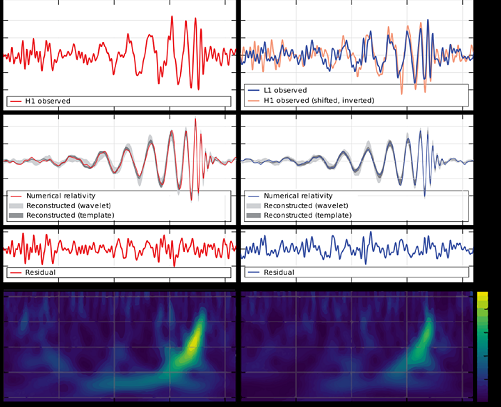Figure 10 - Image of Kungl Vetenskaps Akadamien (Nobel Prize Academy)

The detected gravitational wave was later associated with two black holes in the process of fusion, each with 30 solar masses at a distance of 1.2 billion light years, as suggested in Figure 11.Figure 11

Readers wishing to learn more about interferometry, LASER and the 2017 Nobel Prize can consult:

- Advanced technical documentation from the Nobel Prize Academy -